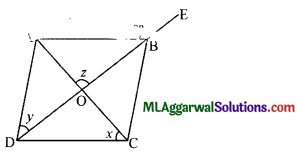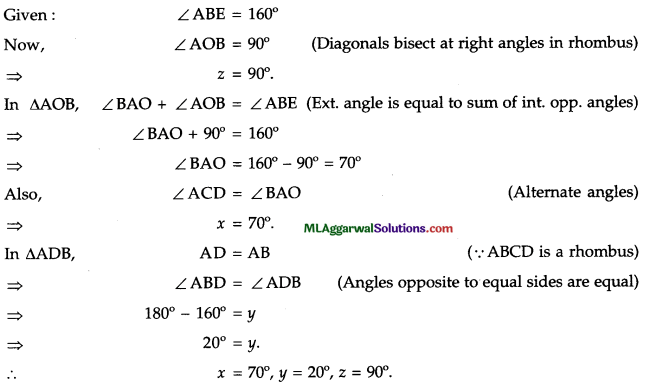# ICSE Class 9 Maths Sample Question Paper 10 with Answers

## ICSE Class 9 Maths Sample Question Paper 10 with Answers

Section – A
(Attempt all questions from this Section)

Question 1.
(a) Evaluate without using tables :
sin 38° sin 52° – cos 38° cos 52°.
sin 38° sin 52° – cos 38° cos 52° = sin 38° sin (90° – 38°) – cos 38° cos (90° – 38°)
= sin 38° cos 38° – cos 38° sin 38°
= 0.(b) In the figure, CD is a diameter which meets the chord AB at E such that AE = BE = 4 cm. If CE = 3 cm, find the radius of the circle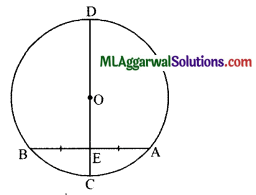Given : CD is a diameter, AE = BE = 4 cm, CE = 3 cm.
∠OEB = 90° (∵ E is the mid-point of BA).
Let the radius OB = OC = r cm.
Then, OE = OC – CE = (r – 3) cm.In ΔOBE, by Pythagoras theorem,
OB2 = OE2 + BE2
⇒ r2 = (r – 3)2 + 42
⇒ r2 = r2 – 2.r.3 + 9 + 16
⇒ 6r = 25
⇒ $$r=\frac{25}{6}=4 \frac{1}{6}$$
The required radius = $$4 \frac{1}{6}$$ cm.

(c) If a = 1 – √3, find the value of $$\left(a-\frac{1}{a}\right)^{3}$$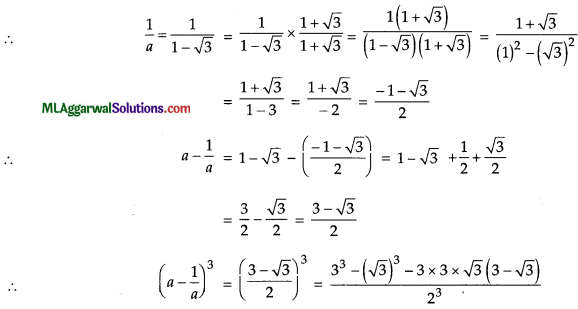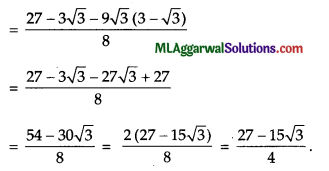Question 2.
If $$a=\frac{1}{a-5}$$,Find
(i) $$a-\frac{1}{a}$$
(ii) $$a+\frac{1}{a}$$
(iii) $$a^{2}-\frac{1}{a^{2}}$$(b) The water bills (in ₹) of 32 houses in a locality are given below.
80, 48, 52, 78, 103, 85, 37, 94, 72, 73, 66, 52, 92, 85, 78, 81, 64, 60, 75, 78, 108, 63, 71, 54, 59, 75, 100, 103, 35, 89, 95, 73.
Construct a frequency distribution table with a class size of 10.
Here, minimum value = 35
Maximum value = 108
Class size =10.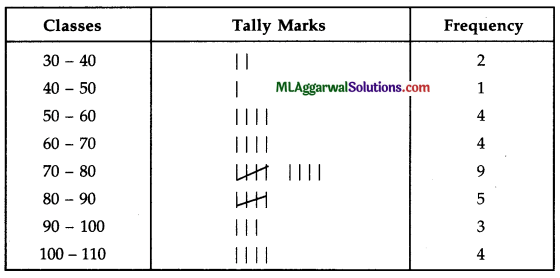(c) Factorize : (a2 – b2) (c2 – d2) – 4 abcd.Question 3.
(a) Show that: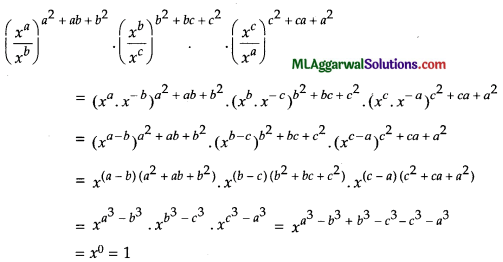(b) In the adjoining figure, AB = CD, CE = BF and ∠ACE = ∠DBF. Prove that:
(i) ΔACE ≅ ΔDBE
(ii) AE = DF.Given: AB = CD,CE = BF and ∠ACE= ∠DBF.
AB = CD
AB + BC = BC + CD (Adding BC on both sides)
⇒ AC = BD.
(i) Now, in ΔACE and ΔDBF
AC = BD (Proved above)
∠ACE = ∠DBF (Given)
CE = BF (Given)
ΔACE ≅ ΔDBF (SAS axiom)
Hence Proved.

(ii) AE = DF (c.p.c.t.)
Hence Proved.(c) If log10y = x, find the value of 102x in terms of y.
Given:
log10 y = x
⇒ y = 10x
Or 10x = y
∴ 102x = (10x)2 = y2

Question 4.
(a) Given, 5 cos A -12 sin A = 0, find the value of $$\frac{\sin A+\cos A}{2 \cos A-\sin A}$$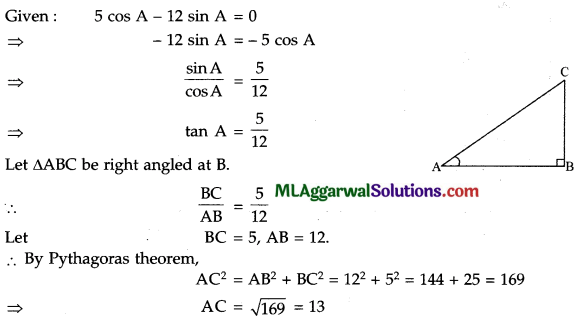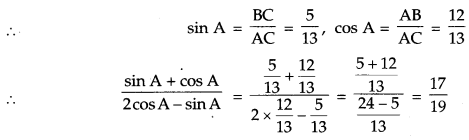(b) Solve by the substitution method :
2x – $$\frac{3}{4}$$y – 3 = 0; 5x – 2y – 7 = 0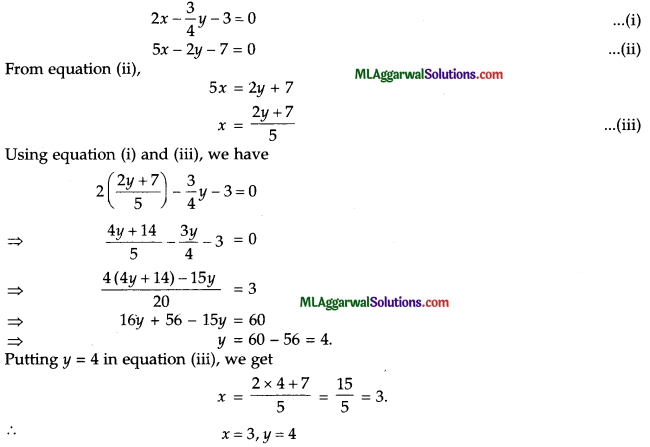(c) What sum of money will amount to ₹ 3630 in 2 years at 10% p. a. compound interest ?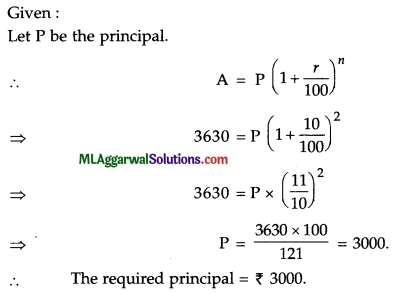Section – B
(Attempt any four questions from this Section)

Question 5.
(a) If x= $$7-4 \sqrt{3}$$,find the value of $$\sqrt{x}+\frac{1}{\sqrt{x}}$$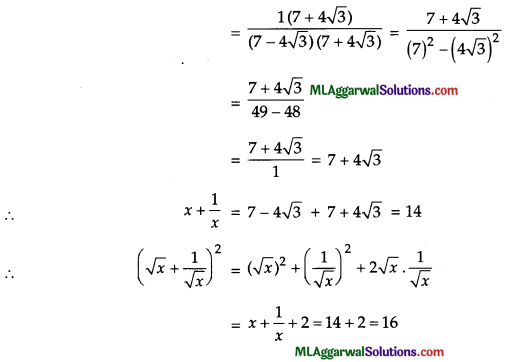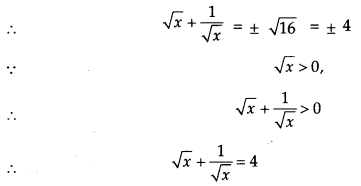(b) In the given figure, X and Y are mid-points of sides AB and AC respectively of ΔABC. If
= 6 cm, AB = 7.4 cm and AC = 6.4 cm, then find the perimeter of the trapezium XBCY.Given : BC = 6 cm, AB = 7.4 cm and AC = 6.4 cm X,
∴ Y are mid-points of AB and AC, respectively
∵ By mid-points theory.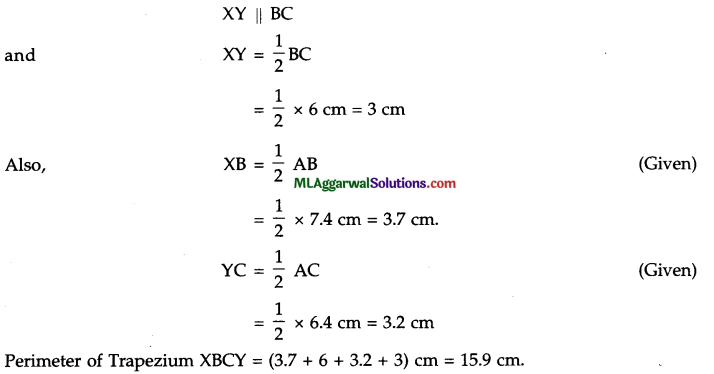(c) The mean weight of 8 students is 46.5 kg. Three more students having weight 41.7 kg, 52.8 kg and 51.8 kg joined the group. What is the new mean weight of the students ?
Given : No. of students = 8, mean weight = 46.5 kg.
∴ Total weight of 8 students = 8 x 46.5 kg = 372 kg
∵ 3 more students joined the group,
∴ Total weight of 11 students = 372 + 41.7 + 52.8 + 51.8 = 518.3 kg.
∴ New mean of 11 students = $$\frac{518.3}{11}$$ = 47.12 kg.Question 6.
(a) Factorize : (x2 – 4x) {x2 – 4x – 1} – 20.
(x2 – 4x) (x2 – 4x – 1) – 20
Let x2 – 4x = a
⇒ a (a – 1) – 20 = a2 – a – 20
⇒ a2 – (5 – 4) a – 20
⇒ a2 -5a + 4a -20
⇒ a (a – 5) + 4 (a – 5)
⇒ (a – 5) (a + 4)
Putting the value of a = x2 – 4x, we get
(x2 – 4x – 5) (x2 – 4x + 4)
= (x2 – 5x + x -5) (x2 – 1. x .2 +22)
= {x {x – 5) + 1 (x – 5)} (x – 2)2
= (x -5) (x + 1) (x – 2)2.

(b) The ratio of incomes of two persons is 9 : 7 and the ratio of their expenditures is 4 : 3. If each of them saves ₹ 200 per month, find their monthly incomes.
The ratio of incomes = 9 : 7 and the ratio of expenditures = 4 : 3
Let their incomes be 9x and 7x and their expenditures be 4y and 3y.
:. Their savings are 9x – 4y and 7x – 3y respectively.
According to the question,
9x – = 200 ———- (i)
7x – = 200 ———- (ii)
Multiplying equation (i) by 3 and equation (ii) by 4, we getTheir incomes are ₹ 1800 and ₹ 1400.(c) The centre of a circle is C (2α -1,3α +1) and it passes through the point A (- 3, – 1). If the diameter of the circle is of length 20 units, find the value (s) of a.
Given points are C(2α – 1, 3α+1) and A(-3,-1)
Diameter 20 units.Question 7.
(a) Insert 3 rational numbers between $$\frac{1}{4}$$ and $$\frac{1}{2}$$.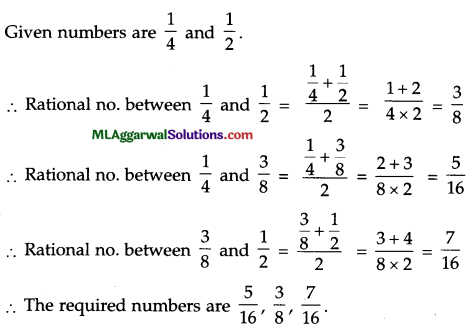(b) In the figure, ABCD is a quadrilateral in which AD = 13 cm, DC = 12 cm, BC = 3 cm and
∠ABD = ∠BCD = 90°. Calculate the length of AB.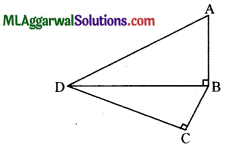Given : AD = 13 cm, DC = 12 cm, BC = 3 cm and ∠ABD = ∠BCD = 90°. (Pythagoras theorem)
In ΔBCD, BD2 = BC2 + CD2
= 32 + 122 = 9 + 144 = 153
In ΔBCD, AD2 = BD2 + AB2
132= 153 + AB2
169 = 153 + AB2
AB2 = 169 – 153 = 16
AB = √16 = 4 cm.

(c) Prove that $$: \frac{1}{1+x^{b-a}+x^{c-a}}+\frac{1}{1+x^{a-b}+x^{c-b}}+\frac{1}{1+x^{b-c}+x^{a-c}}=1$$Question 8.
(a) A sum amounts to ₹ 9680 in 2 years and to ₹ 10648 in 3 years compounded annually. Find the sum and the rate of interest per annum.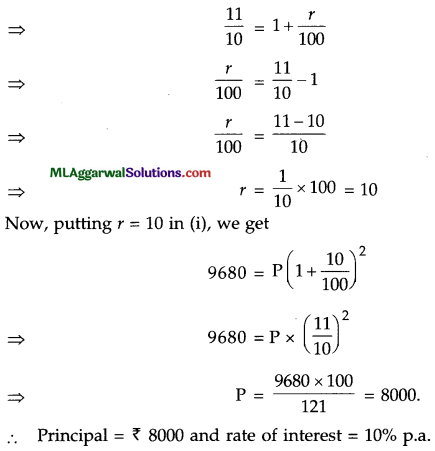(b) A triangle is formed by the lines x + 2y – 3 = 0, 3x – 2y + 7 = 0 and y + 1=0. Find graphically :
(i) The coordinates of vertices of the triangle.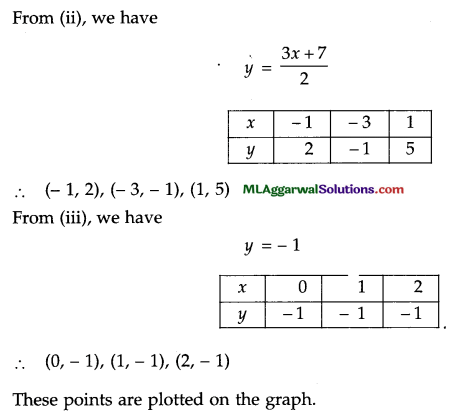Let the lines intersect at the points A, B and C.
(i) The coordinates of vertices of triangle are A (- 1, 2), B (- 3, – 1) and C (5, – 1).
(ii) From A, draw AM ⊥ BC.
Area of Δ ABC
= $$\frac{1}{2}$$ × BC × AM
= $$\frac{1}{2}$$ × 8 × 3
= 12 sq. units.(c) Solve : $$\frac{8}{x+3}-\frac{3}{2-x}=2$$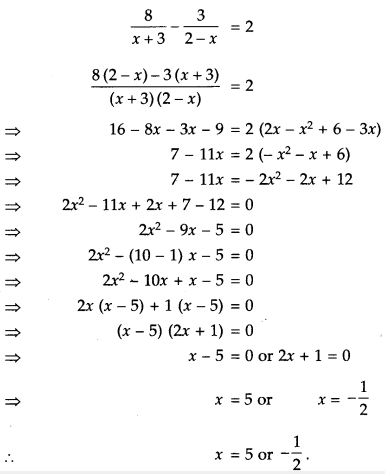Question 9.
(a) In the figure, ABCD is a parallelogram and P is any point on BC. Prove that area of ΔABP + area of ΔDPC = area of ΔAPD.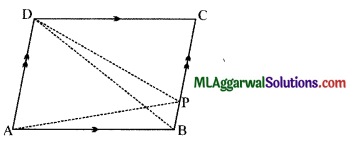Given : ABCD is a parallelogram
We know, triangles on same base and between same parallels are equal.
∴ Area of AAPD = Area of AABD ……. (i)
and Area of AABP = Area of ABDP ……. (ii)
Also, since diagonal of a parallelogram divides it into two triangles of equal area
∴ Area of ΔBCD = Area of ΔABD …… (iii)
⇒ Area of ΔBDP + Area of ΔDPC = Area of ΔABD
⇒ Area of ΔABP + Area of ΔDPC = Area of ΔAPD [Using (i) and (ii)]
Hence Proved.

(b) Factorize : 1+a+b+c+ab+bc+ca+abc.
1+a+b+c+ab+bc+ca+abc=(1+a)+(b+ab)+(c+ca)+(bc+abc)
=(1+a)+b(1+a)+c(1+a)+bc(1+a)
=(1+a)(1+b+c+bc)
=(1+a)((1+b)+c(1+b)
= (1+ a) (1+ b) (1+ c).(c) The area of cross-section of a pipe is 5.4 cm2 and water is pumped out of it at the rate of 27 km/h. Find in litres the volume of water which flows out of the pipe in one minute.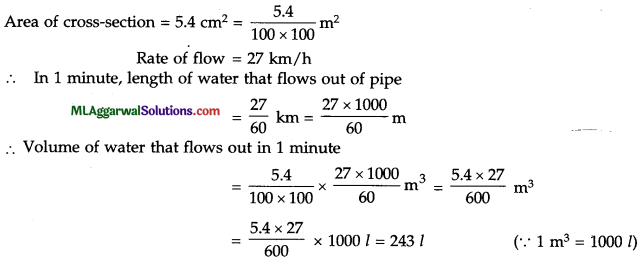Question 10.
(a) If 4 cos2 x° – 1 = 0 and 0 ≤ x°≤ 90°,find the value of cos2 x° sin2 x°.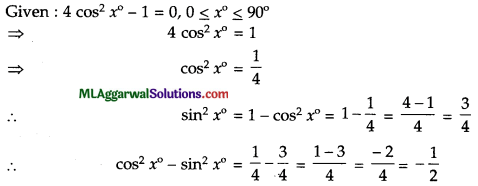(b) Draw the parallelogram ABCD in which AB = BC = 4.8 cm and AC = 7.5 cm. Find the angle between the two diagonals. What special name can you give it to this parallelogram?
Given : AB = BC = 4.8 cm, AC = 7.5cm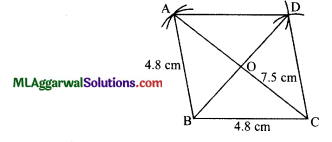Steps of construction:
(1) Draw BC = 4.8 cm.
(2) From B and C, draw arcs of length 4.8 cm and 7.5 cm, respectively which intersect at A.
(3) From A and C, draw arcs each of length 4.8 cm which intersect at D.
(4) Join BA, AD and CD to complete the required parallelogram.
(5) Join AC and BD which intersect at O.
∴ ∠AOD=90°
The special name of this parallelogram is rhombus as all sides are equal.(c) Elavuate $$\frac{5^{10+n} \times 25^{3 n-4}}{5^{7 n}}$$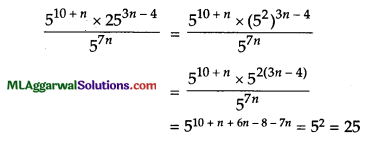Question 11.
(a) Prove that: $$x^{\log y-\log z} \times y^{\log z-\log x} \times z^{\log x-\log y}=1$$(b) In the figure, ABCD is a rhombus in which the diagonal DB is produced to E. If ∠ABE = 160°, then find x, y and z.